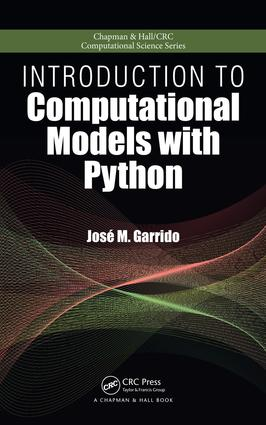# Introduction to Computational Models with Python

## 1st Edition

Chapman and Hall/CRC

466 pages | 90 B/W Illus.

##### Purchasing Options:\$ = USD
Hardback: 9781498712033
pub: 2015-09-04
SAVE ~\$19.59
\$97.95
\$78.36
x
eBook (VitalSource) : 9780429183263
pub: 2015-08-28
from \$46.98

FREE Standard Shipping!

### Description

Introduction to Computational Models with Python explains how to implement computational models using the flexible and easy-to-use Python programming language. The book uses the Python programming language interpreter and several packages from the huge Python Library that improve the performance of numerical computing, such as the Numpy and Scipy modules. The Python source code and data files are available on the author’s website.

The book’s five sections present:

1. An overview of problem solving and simple Python programs, introducing the basic models and techniques for designing and implementing problem solutions, independent of software and hardware tools
2. Programming principles with the Python programming language, covering basic programming concepts, data definitions, programming structures with flowcharts and pseudo-code, solving problems, and algorithms
3. Python lists, arrays, basic data structures, object orientation, linked lists, recursion, and running programs under Linux
4. Implementation of computational models with Python using Numpy, with examples and case studies
5. The modeling of linear optimization problems, from problem formulation to implementation of computational models

This book introduces the principles of computational modeling as well as the approaches of multi- and interdisciplinary computing to beginners in the field. It provides the foundation for more advanced studies in scientific computing, including parallel computing using MPI, grid computing, and other methods and techniques used in high-performance computing.

Problem Solving

Problem Solving and Computing

INTRODUCTION

COMPUTER PROBLEM SOLVING

ELEMENTARY CONCEPTS

DEVELOPING COMPUTATIONAL MODELS

TEMPERATURE CONVERSION

AREA AND PERIMETER OF A CIRCLE

CATEGORIES OF COMPUTATIONAL MODELS

GENERAL PROCESS OF SOFTWARE DEVELOPMENT

MODULAR DESIGN

PROGRAMMING LANGUAGES

PRECISION, ACCURACY, AND ERRORS

Simple Python Programs

INTRODUCTION

COMPUTING WITH PYTHON

PROGRAMS

DATA DEFINITIONS

SIMPLE PYTHON PROGRAMS

A SIMPLE PROBLEM: TEMPERATURE CONVERSION

DISTANCE BETWEEN TWO POINTS

GENERAL STRUCTURE OF A PYTHON PROGRAM

SIMPLE FUNCTIONS

Basic Programming Principles with Python

Modules and Functions

INTRODUCTION

MODULAR DECOMPOSITION

FUNCTIONS

CATEGORIES OF FUNCTIONS

BUILT-IN MATHEMATICAL FUNCTIONS

Program Structures

INTRODUCTION

ALGORITHMS

IMPLEMENTING ALGORITHMS

ALGORITHM DESCRIPTION

DESIGN STRUCTURES

COMPUTING AREA AND CIRCUMFERENCE

The Selection Program Structure

INTRODUCTION

CONDITIONAL EXPRESSIONS

THE SELECTION STRUCTURE

A COMPUTATIONAL MODEL WITH SELECTION

MULTI-LEVEL SELECTION

The Repetition Program Structure

INTRODUCTION

REPETITION WITH THE WHILE-LOOP

REPEAT-UNTIL LOOP

FOR-LOOP STRUCTURE

Data Structures, Object Orientation, and Recursion

Python Lists, Strings, and Other Data Sequences

INTRODUCTION

LISTS

TEMPERATURE CONVERSION PROBLEM

LIST COMPREHENSIONS

LISTS OF LISTS

TUPLES

DICTIONARIES

STRINGS

SIMPLE NUMERICAL APPLICATIONS USING LISTS

Object Orientation

INTRODUCTION

OBJECTS IN THE PROBLEM DOMAIN

DEFINING CLASSES

DESCRIBING OBJECTS

INTERACTION BETWEEN TWO OBJECTS

DESIGN WITH CLASSES

Object-Oriented Programs

INTRODUCTION

PROGRAMS

DEFINITION OF CLASSES

CLASS DEFINITIONS IN PYTHON

CREATING AND MANIPULATING OBJECTS

COMPLETE PROGRAM WITH A CLASS

SCOPE OF VARIABLES

CLASS HIERARCHY WITH INHERITANCE

DEFINING CLASSES WITH INHERITANCE

INTRODUCTION

NODES AND LINKED LISTS

LINKED LISTS WITH TWO ENDS

STACKS AND QUEUES DATA STRUCTURES

Recursion

INTRODUCTION

RECURSIVE APPROACH TO PROBLEM SOLVING

RECURSIVE DEFINITION OF FUNCTIONS

ANALYZING RECURSION

Fundamental Computational Models with Python

Computational Models with Arithmetic Growth

INTRODUCTION

MATHEMATICAL MODELING

MODELS WITH ARITHMETIC GROWTH

USING THE PYTHON LANGUAGE AND NUMPY

PRODUCING THE CHARTS OF THE MODEL

VALIDATION OF A MODEL

FILE I/O

Computational Models with Quadratic Growth

INTRODUCTION

DIFFERENCES OF THE DATA

DIFFERENCE EQUATIONS

FUNCTIONAL EQUATIONS

EXAMPLES OF QUADRATIC MODELS

Models with Geometric Growth

INTRODUCTION

BASIC CONCEPTS

FUNCTIONAL EQUATIONS IN GEOMETRIC GROWTH

Computational Models with Polynomial Growth

INTRODUCTION

GENERAL FORMS OF POLYNOMIAL FUNCTIONS

THE polynomial MODULE OF THE numpy PACKAGE

EVALUATION OF POLYNOMIAL FUNCTIONS

SOLVING POLYNOMIAL FUNCTIONS

Empirical Models with Interpolation and Curve Fitting

INTRODUCTION

INTERPOLATION

CURVE FITTING

MODELING THE HEAT CAPACITY OF CARBON DIOXIDE

Using Arrays with Numpy

INTRODUCTION

VECTORS AND OPERATIONS

VECTOR PROPERTIES AND CHARACTERISTICS

USING ARRAYS IN PYTHON WITH NUMPY

SIMPLE VECTOR OPERATIONS

Models with Matrices and Linear Equations

INTRODUCTION

MATRICES

MATRIX MANIPULATION WITH NUMPY

SOLVING SYSTEMS OF LINEAR EQUATIONS

INDUSTRIAL MIXTURES IN MANUFACTURING

Introduction to Models of Dynamical Systems

INTRODUCTION

AVERAGE AND INSTANTANEOUS RATE OF CHANGE

THE FREE-FALLING OBJECT

DERIVATIVE OF A FUNCTION

NUMERICAL INTEGRATION

WORK PRODUCED IN A PISTON WITH AN IDEAL GAS

DIFFERENTIAL EQUATIONS

MODELS OF DYNAMICAL SYSTEMS

FORMULATING SIMPLE EXAMPLES

SOLUTION OF DIFFERENTIAL EQUATIONS

Linear Optimization Models

Linear Optimization Modeling

INTRODUCTION

GENERAL FORM OF A LINEAR OPTIMIZATION MODEL

THE SIMPLEX ALGORITHM

DESCRIPTION OF THE SIMPLEX ALGORITHM

FORMULATION OF LINEAR OPTIMIZATION MODELS

EXAMPLE PROBLEMS

Solving Linear Optimization Models

INTRODUCTION

LINEAR OPTIMIZATION MODELS WITH PYTHON

MODELING WITH PYOMO

MODELING WITH PULP

SOFTWARE LINEAR OPTIMIZATION SOLVERS

SHORT LIST OF OPTIMIZATION SOLVERS

Sensitivity Analysis and Duality

INTRODUCTION

SENSITIVITY ANALYSIS

DUALITY

Transportation Models

INTRODUCTION

MODEL OF A TRANSPORTATION PROBLEM

TRANSPORTATION CASE STUDY 1

UNBALANCED PROBLEM: CASE STUDY 2

UNBALANCED PROBLEM: CASE STUDY 3

TRANSSHIPMENT MODELS

TRANSSHIPMENT PROBLEM: CASE STUDY 4

ASSIGNMENT PROBLEMS 7

ASSIGNMENT PROBLEM: CASE STUDY 5

Network Models

INTRODUCTION

GRAPHS

SHORTEST PATH PROBLEM

SHORTEST PATH: CASE STUDY 1

MAXIMUM FLOW PROBLEMS

CRITICAL PATH METHOD

REDUCING THE TIME TO COMPLETE A PROJECT

Integer Linear Optimization Models

INTRODUCTION

MODELING WITH INTEGER VARIABLES

APPLICATIONS OF INTEGER LINEAR OPTIMIZATION

INTEGER LINEAR OPTIMIZATION: CASE STUDY 1

INTEGER LINEAR OPTIMIZATION: CASE STUDY 2

A Summary and Exercises appear at the end of each chapter.

### About the Author

José M. Garrido is a professor in the Department of Computer Science at Kennesaw State University. Dr. Garrido is the author of several books and numerous research papers. His research interests include software development, operating systems, computational modeling, object-oriented simulation, and system formal specification.

### Subject Categories

##### BISAC Subject Codes/Headings:
COM000000
COMPUTERS / General
COM012040
COMPUTERS / Programming / Games
MAT000000
MATHEMATICS / General